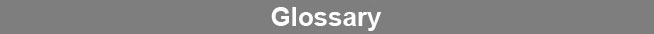Select Page### Variance

The variance of a parameter pool is a measurement of the variability of the pool. It is determined by squaring the deviations of each value about the mean and dividing by one less than the number of individuals in the pool. The variance is the square of the standard deviation.www.brendan.com